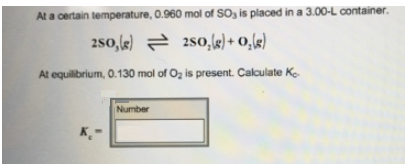# Problem: At a certain temperature, O.960 mol of SO3 is placed in a 3.00-L container. 2SO3 (g) ⇌ 2SO2 (g) + O2 (g) At equilibrium, 0.130 mol of O2 is present. Calculate Kc.

###### FREE Expert Solution
82% (438 ratings)###### Problem Details

At a certain temperature, O.960 mol of SO3 is placed in a 3.00-L container.

2SO3 (g) ⇌ 2SO2 (g) + O2 (g)

At equilibrium, 0.130 mol of O2 is present. Calculate Kc